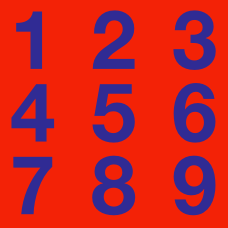Number Theory

# Number Theory Warmups: Level 2 Challenges

Magician Mike Michael claims to know two positive whole numbers that multiply to 1000, neither of which contain the digit 0.

What is the sum of these 2 numbers?

$\sqrt { { 17 }^{ 2 }+{ 17 }^{ 2 }+{ 17 }^{ 2 }+...+{ 17 }^{ 2 }+{ 17 }^{ 2 }+{ 17 }^{ 2 } }\\ ={ 17 }^{ 2 }+{ 17 }^{ 2 }+{ 17 }^{ 2 }$

How many times should ${17}^{2}$ appear under the square root sign for the equation above to be true?

$\Large x! = \frac { (7!)!}{7!}, \ \ \ \ \ \ \ x = \ ?$Can an even number, divided by another even number, times another even number ever equal an odd number?

If "yes," then find three numbers that work.
If "no," then why not?

Note that the three even numbers can be different numbers.

Is 87985 divisible by 7?

×